Pyritohedron

When you fold a dodecahedron into a cube, inside the cube there is an empty space.A Cube can be inscribed in a Dodecahedron. A Dodecahedron can be seen as a cube with six 'roofs'. You can fold a dodecahedron into a cube.We can fill this empty space with a polyhedron that is a kind of pyritohedron, that is to say, this polyhedron is an irregular dodecahedron composed of identical irregular pentagons. In this case is a concave pyritohedron an is called concave pyritohedral dodecahedron.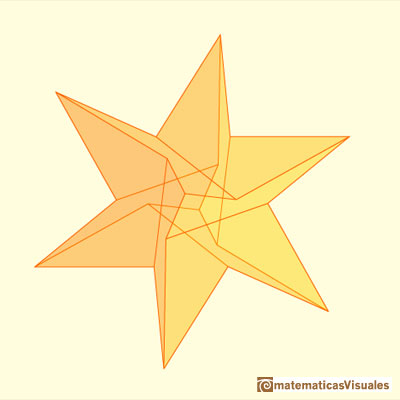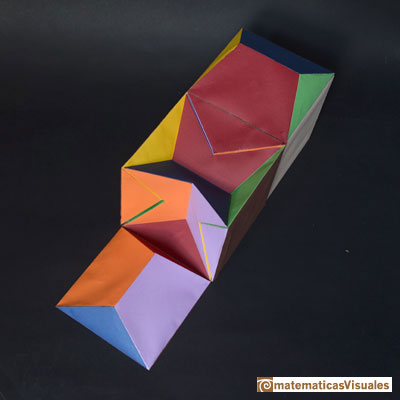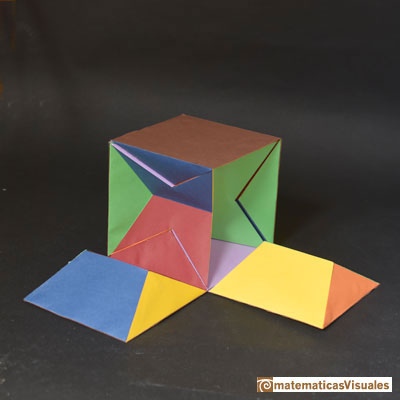We encourage you to build your own pyritohedron: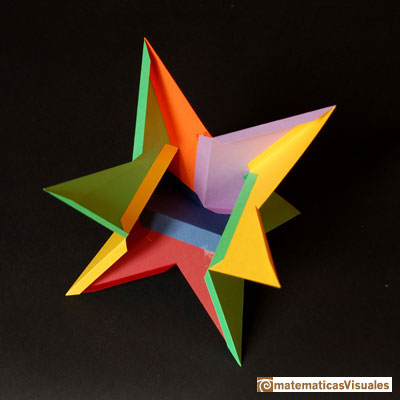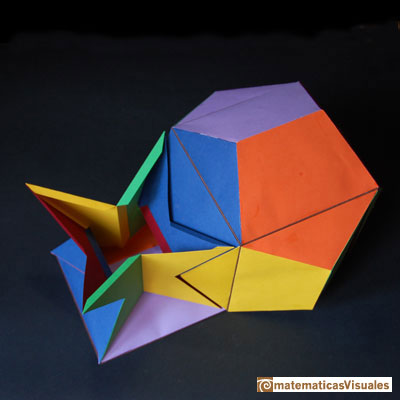We can build this pyritohedron using Zome:Eight vertices are the vertices of a cube:In the interior, twelve vertices are in three golden rectangles: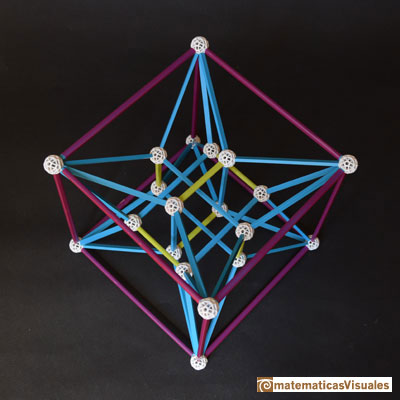The three golden rectangles in three different colors:These twelve vertices are the vertices of an icosahedron: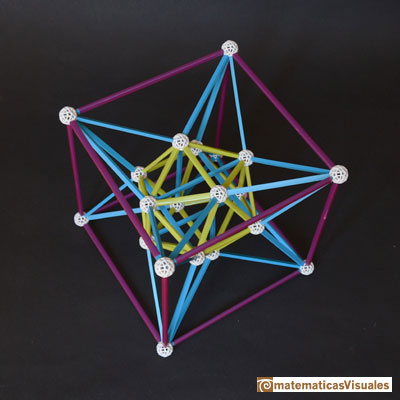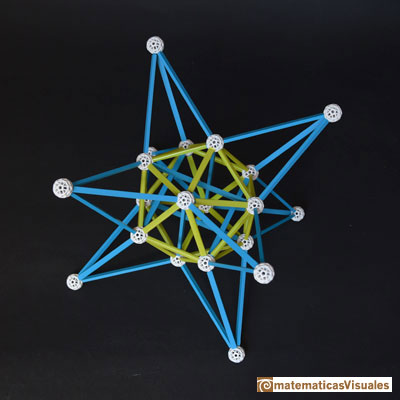To calculate the volume of this pyritohedron of side length 1 you should remember some properties of the golden ratio, and it is very similar to the calculation of the volume of a dodecahedron. The roof that was outside the cube it is now inside the cube.The diagonal of a regular pentagon are in golden ratio to its sides and the point of intersection of two diagonals of a regular pentagon are said to divide each other in the golden ratio or 'in extreme and mean ratio'.One eighth of a regular dodecahedon of edge 2 has the same volume as a dodecahedron of edge 1.

The volume of this pyritohedron is the volume of a cube minus six times the volume of a roof.

Remember the volume of the cube:

And the volume of one roof is:

Then the volume of this pyritohedron is:

REFERENCES

Zome is a wonderful tool to build polyhedra.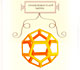Leonardo da Vinci made several drawings of polyhedra for Luca Pacioli's book 'De divina proportione'. Here we can see an adaptation of the dodecahedron.Some properties of this platonic solid and how it is related to the golden ratio. Constructing dodecahedra using different techniques.One eighth of a regular dodecahedon of edge 2 has the same volume as a dodecahedron of edge 1.The first drawing of a plane net of a regular dodecahedron was published by Dürer in his book 'Underweysung der Messung' ('Four Books of Measurement'), published in 1525 .The diagonal of a regular pentagon are in golden ratio to its sides and the point of intersection of two diagonals of a regular pentagon are said to divide each other in the golden ratio or 'in extreme and mean ratio'.You can draw a regular pentagon given one of its sides constructing the golden ratio with ruler and compass.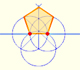In his book 'Underweysung der Messung' Durer draw a non-regular pentagon with ruler and a fixed compass. It is a simple construction and a very good approximation of a regular pentagon.From Euclid's definition of the division of a segment into its extreme and mean ratio we introduce a property of golden rectangles and we deduce the equation and the value of the golden ratio.A golden rectangle is made of an square and another golden rectangle.The golden spiral is a good approximation of an equiangular spiral.